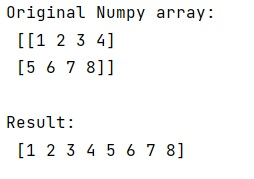# Python numpy.reshape() Method: What does -1 mean in it?

numpy.reshape(): In this tutorial, we will learn about the numpy.reshape() method, and what does -1 mean in this method. By Pranit Sharma Last updated : May 23, 2023

NumPy is an abbreviated form of Numerical Python. It is used for different types of scientific operations in python. Numpy is a vast library in python which is used for almost every kind of scientific or mathematical operation. It is itself an array which is a collection of various methods and functions for processing the arrays.

## numpy.reshape() Method

The numpy.reshape() method is used to give a new shape to an array without actually changing its data.

### Syntax

```numpy.reshape(a, newshape, order='C')
```

### Parameter(s)

• a: array to be reshaped.
• newshape: int value of a tuple of int values.
• order: Reads the elements of the given array using this index order, and places the elements into the reshaped array using this index order.

### Return Value

The numpy.reshape() method returns a reshaped array of ndarray type.

## What does -1 mean in it?

We know that a two-dimensional array can be reshaped into a one-dimensional array. Numpy provides a method called reshape() where if we pass -1 as an arguement, it will convert a two-dimensional array into a one-dimensional array.

Let us understand with the help of an example,

## Example of numpy.reshape() Method in Python by passing -1 in it

```# Import numpy
import numpy as np

# Creating a Numpy array
arr = np.array([[1, 2, 3, 4], [5, 6, 7, 8]])

# Display original numpy array
print("Original Numpy array:\n",arr,"\n")

# Using -1 arguement for reshaping
# this array
res = arr.reshape(-1)

# Display result
print("Result:\n",res)
```

### Output